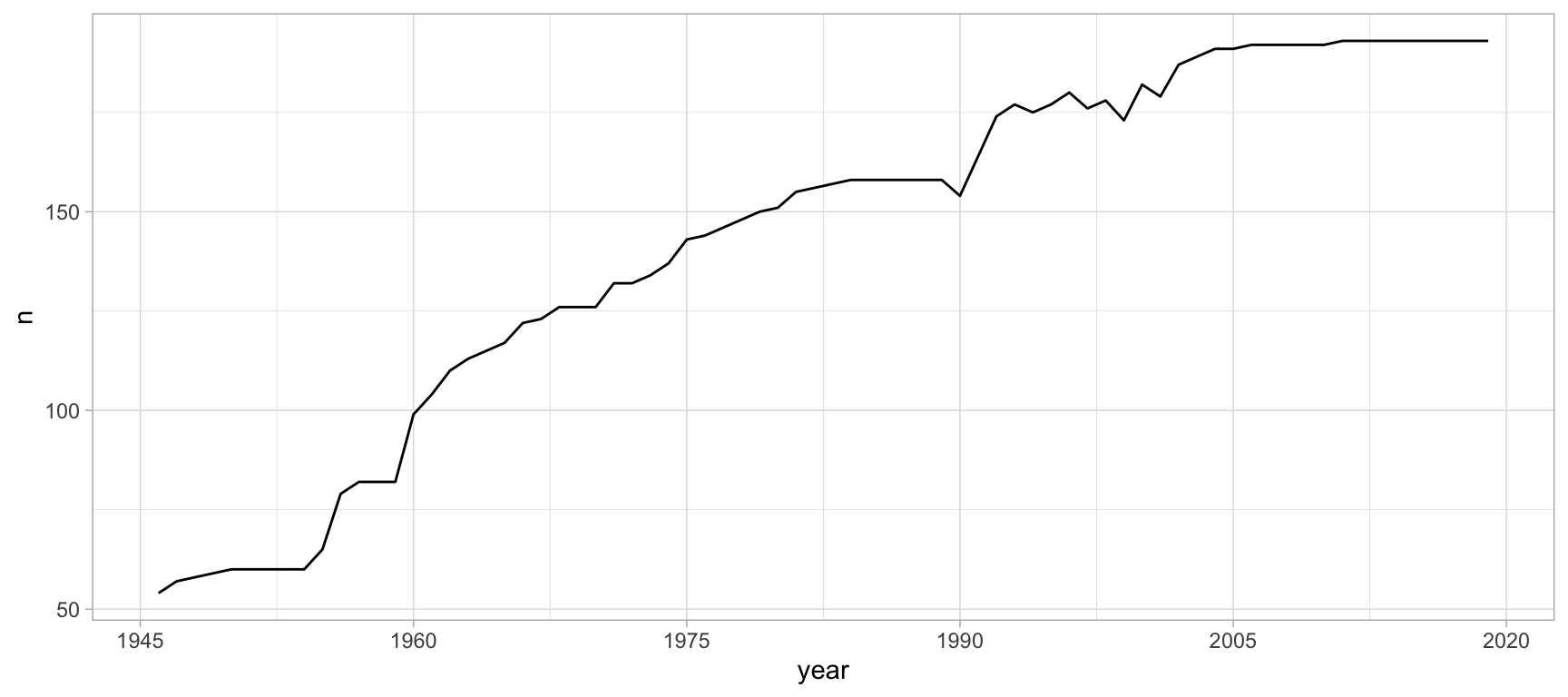# Introduction

## Endogenous state theory

Counting the number of countries in the UN.

``````library(unvotes)
library(tidyverse)
library(lubridate)
mutate(year = year(date)) %>%
count(country, country_code, year) %>%
count(year) %>%
ggplot(aes(x = year, y = n)) +
geom_line() +
scale_x_continuous(breaks = seq(1945, 2020, 15)) +
scale_y_continuous(breaks = seq(50, 200, 50)) +
theme_light()``````Figure 1: Number of countries in the UN

Previous
Next